# Scatter blocks inside a polyline or some kind of spray tool for ACAD ? ...## Recommended PostsI need some kind of scatter tool or spray tool, that would spread some blocks/trees over an area, or closed polyline ...

I've seen there was once a routine or addon called Sketch++ or skpp, but it's not available for download anymore ... says "not free anymore" but I cant even find the commercial version of it

I want to use it to make some urban planning drawings more "alive" ... I'm working on a little settlement plan that's in the middle of a large forest, and would love to somehow "randomize" the trees, their position and scale ...

I know there are tons of plugins for 3dsmax for example, that spread or spray/paint selected 3d objects, with many options regarding distance, scale, density, ...

Is there somethig similar for Autocad, or could someone give it a try if its not too complicated ?

Cheers,

Miroslav

• Replies 20
• Created

#### Top Posters In This Topic

•5

•4

•2

•2

#### Top Posters In This Topic

•marko_ribar 5 posts

•rlx 4 posts

•gashaglava 2 posts

•cupax 2 posts

#### Popular PostsCheck this program... (you have to be logged in...)   https://www.theswamp.org/index.php?topic=48113.msg531626#msg531626   Regards, M.R.The original would have been 1e-8 since all occurrences of "8)" were removed from all code posts across the forum when the forum software was "upgraded".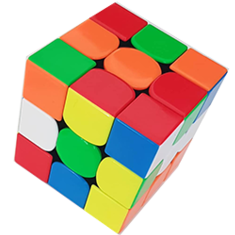Interesting conceptgetdist

getint

some kind of code

insert

exit

Maybe or maybe not.##### Share on other sitesHere, try this :

```(defun rnd (/ modulus multiplier increment rand)
(if (not seed)
(setq seed (getvar "DATE"))
)
(setq modulus    65536
multiplier 25173
increment  13849
seed  (rem (+ (* multiplier seed) increment) modulus)
rand     (/ seed modulus)
)
)

(defun GroupByNum ( lst n / r)
(if lst
(cons
(reverse (repeat n (setq r (cons (car lst) r) lst (cdr lst)) r))
(GroupByNum lst n)
)
)
)

(defun ptinsideent ( pt ent / msp ptt lin int a b tst result )
(setq ptt (vlax-curve-getclosestpointto ent pt))
(setq lin (vla-addline msp (vlax-3d-point pt) (vlax-3d-point ptt)))
(setq int (GroupByNum (vlax-invoke (if (eq (type ent) 'ENAME) (vlax-ename->vla-object ent)) 'intersectwith lin acExtendBoth) 3))
(setq a (angle pt ptt))
(foreach p int
(setq b (angle pt p))
(if (equal a b 1e- (setq tst (cons T tst)) (setq tst (cons nil tst)))
)
(vla-delete lin)
(if (eval (cons 'and tst)) (setq result nil) (setq result T))
result
)

(defun c:populate ( / BNAME DX DXX DY DYY ENT ENTA MAXPOINT MAXPT MINPOINT MINPT MSP NO PT SCF SCFF SCFFMIN ) (vl-load-com)
(setq ent (car (entsel "\nPick 2D closed entity without arcs")))
(while (eq (cdr (assoc 70 (entget ent))) 0)
(prompt "\nPicked entity is open, please pick closed one")
(setq ent (car (entsel "\nPick 2D closed entity without arcs")))
)
(setq entA (vlax-ename->vla-object ent))
(vla-getboundingbox entA 'minpoint 'maxpoint)
(setq
minpt (vlax-safearray->list minpoint)
maxpt (vlax-safearray->list maxpoint)
)
(setq dx (- (car maxpt) (car minpt)))
(initget 6)
(setq no (getint "\nInput number of blocks to populate : "))
(setq bname "")
(while (not (tblsearch "BLOCK" bname))
(setq bname (getstring T "\nInput name of block to populate (CASE UNSENSITIVE) : "))
)
(initget 6)
(setq scf (getreal "\nInput max. scale factor for block insertion <1.0> : "))
(if (null scf) (setq scf 1.0))
(initget 6)
(setq scfmin (getreal "\nInput min. scale factor for block insertion <1.0> : "))
(if (null scfmin) (setq scfmin 1.0))
(while (> no 0)
(setq dxx (* dx (rnd)))
(setq dyy (* dy (rnd)))
(setq pt (list (+ (car minpt) dxx) (+ (cadr minpt) dyy) 0.0))
(if (and (eq scfmin 1.0) (eq scf 1.0)) (setq scff 1.0) (setq scff (+ scfmin (* (- scf scfmin) (rnd)))))
(if (ptinsideent pt ent)
(progn
(setq no (1- no))
(vla-insertblock msp (vlax-3d-point pt) bname scff scff scff (* 2 pi (rnd)))
)
)
)
(princ)
)
```

M.R.

Hope this should help...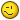Edited by marko_ribar
##### Share on other sitesEXACTLY !

Many, many thanx !

Cheers

##### Share on other sitesHere is revision (changed ptinsideent sub-function)... Now routine can be applied and for closed 2D entities with arcs...

```(defun rnd (/ modulus multiplier increment rand)
(if (not seed)
(setq seed (getvar "DATE"))
)
(setq modulus    65536
multiplier 25173
increment  13849
seed  (rem (+ (* multiplier seed) increment) modulus)
rand     (/ seed modulus)
)
)

(defun GroupByNum ( lst n / r)
(if lst
(cons
(reverse (repeat n (setq r (cons (car lst) r) lst (cdr lst)) r))
(GroupByNum lst n)
)
)
)

(defun ptinsideent ( pt ent / msp ptt lin int a b tst result )
(setq ptt (vlax-curve-getclosestpointto ent pt))
(setq lin (vla-addline msp (vlax-3d-point pt) (vlax-3d-point ptt)))
(setq int (GroupByNum (vlax-invoke (if (eq (type ent) 'ENAME) (vlax-ename->vla-object ent)) 'intersectwith lin acExtendBoth) 3))
(setq a (angle pt ptt))
(setq b (angle pt (car (vl-remove ptt int))))
(if (or (equal a b 1e- (> (length int) 2)) (setq tst (cons T tst)) (setq tst (cons nil tst)))
(vla-delete lin)
(if (eval (cons 'and tst)) (setq result nil) (setq result T))
result
)

(defun c:populate ( / BNAME DX DXX DY DYY ENT ENTA MAXPOINT MAXPT MINPOINT MINPT MSP NO PT SCF SCFF SCFFMIN ) (vl-load-com)
(setq ent (car (entsel "\nPick 2D closed entity")))
(while (eq (cdr (assoc 70 (entget ent))) 0)
(prompt "\nPicked entity is open, please pick closed one")
(setq ent (car (entsel "\nPick 2D closed entity")))
)
(setq entA (vlax-ename->vla-object ent))
(vla-getboundingbox entA 'minpoint 'maxpoint)
(setq
minpt (vlax-safearray->list minpoint)
maxpt (vlax-safearray->list maxpoint)
)
(setq dx (- (car maxpt) (car minpt)))
(initget 6)
(setq no (getint "\nInput number of blocks to populate : "))
(setq bname "")
(while (not (tblsearch "BLOCK" bname))
(setq bname (getstring T "\nInput name of block to populate (CASE UNSENSITIVE) : "))
)
(initget 6)
(setq scf (getreal "\nInput max. scale factor for block insertion <1.0> : "))
(if (null scf) (setq scf 1.0))
(initget 6)
(setq scfmin (getreal "\nInput min. scale factor for block insertion <1.0> : "))
(if (null scfmin) (setq scfmin 1.0))
(while (> no 0)
(setq dxx (* dx (rnd)))
(setq dyy (* dy (rnd)))
(setq pt (list (+ (car minpt) dxx) (+ (cadr minpt) dyy) 0.0))
(if (and (eq scfmin 1.0) (eq scf 1.0)) (setq scff 1.0) (setq scff (+ scfmin (* (- scf scfmin) (rnd)))))
(if (ptinsideent pt ent)
(progn
(setq no (1- no))
(vla-insertblock msp (vlax-3d-point pt) bname scff scff scff (* 2 pi (rnd)))
)
)
)
(princ)
)
```

Regards, M.R.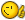Edited by marko_ribar
##### Share on other sitesAnother slower version, but spreading is better...

```(defun rnd (/ modulus multiplier increment rand)
(if (not seed)
(setq seed (getvar "DATE"))
)
(setq modulus    65536
multiplier 25173
increment  13849
seed  (rem (+ (* multiplier seed) increment) modulus)
rand     (/ seed modulus)
)
)

(defun ptinsideent ( pt ent / ss result )
(setq ss (ssget "_X"))
(vl-cmdf "_.-boundary" "a" "b" "n" ent "" "" pt "")
(if (> (sslength (ssget "_X")) (sslength ss)) (progn (setq result T) (entdel (entlast))) (setq result nil))
result
)

(defun c:populate ( / BNAME DX DXX DY DYY ENT ENTA MAXPOINT MAXPT MINPOINT MINPT MSP NO PT SCF SCFF SCFFMIN ) (vl-load-com)
(setq ent (car (entsel "\nPick 2D closed entity")))
(while (eq (cdr (assoc 70 (entget ent))) 0)
(prompt "\nPicked entity is open, please pick closed one")
(setq ent (car (entsel "\nPick 2D closed entity")))
)
(setq entA (vlax-ename->vla-object ent))
(vla-getboundingbox entA 'minpoint 'maxpoint)
(setq
minpt (vlax-safearray->list minpoint)
maxpt (vlax-safearray->list maxpoint)
)
(setq dx (- (car maxpt) (car minpt)))
(initget 6)
(setq no (getint "\nInput number of blocks to populate : "))
(setq bname "")
(while (not (tblsearch "BLOCK" bname))
(setq bname (getstring T "\nInput name of block to populate (CASE UNSENSITIVE) : "))
)
(initget 6)
(setq scf (getreal "\nInput max. scale factor for block insertion <1.0> : "))
(if (null scf) (setq scf 1.0))
(initget 6)
(setq scfmin (getreal "\nInput min. scale factor for block insertion <1.0> : "))
(if (null scfmin) (setq scfmin 1.0))
(while (> no 0)
(setq dxx (* dx (rnd)))
(setq dyy (* dy (rnd)))
(setq pt (list (+ (car minpt) dxx) (+ (cadr minpt) dyy) 0.0))
(if (and (eq scfmin 1.0) (eq scf 1.0)) (setq scff 1.0) (setq scff (+ scfmin (* (- scf scfmin) (rnd)))))
(if (ptinsideent pt ent)
(progn
(setq no (1- no))
(vla-insertblock msp (vlax-3d-point pt) bname scff scff scff (* 2 pi (rnd)))
)
)
)
(princ)
)
```

Regards, M.R.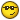##### Share on other sitesFINAL VERSION

(CAN BE APPLIED ON ENTITY WITH ARCS - MUCH FASTER THAN -BOUNDARY VERSION)

```(defun rnd (/ modulus multiplier increment rand)
(if (not seed)
(setq seed (getvar "DATE"))
)
(setq modulus    65536
multiplier 25173
increment  13849
seed  (rem (+ (* multiplier seed) increment) modulus)
rand     (/ seed modulus)
)
)

(defun GroupByNum ( lst n / r)
(if lst
(cons
(reverse (repeat n (setq r (cons (car lst) r) lst (cdr lst)) r))
(GroupByNum lst n)
)
)
)

(defun ptonline ( pt pt1 pt2 / vec12 vec1p d result )
(setq vec12 (mapcar '- pt2 pt1))
(setq vec12 (reverse (cdr (reverse vec12))))
(setq vec1p (mapcar '- pt pt1))
(setq vec1p (reverse (cdr (reverse vec1p))))
(setq vec2p (mapcar '- pt2 pt))
(setq vec2p (reverse (cdr (reverse vec2p))))
(setq d (distance '(0.0 0.0) vec12) d1 (distance '(0.0 0.0) vec1p) d2 (distance '(0.0 0.0) vec2p))
(if (equal d (+ d1 d2) 1e- (setq result T) (setq result nil))
result
)

(defun ptinsideent ( pt ent / msp ptt xlin int k kk tst result )
(setq ptt (vlax-curve-getclosestpointto ent pt))
(setq xlin (vla-addxline msp (vlax-3d-point pt) (vlax-3d-point ptt)))
(setq int (GroupByNum (vlax-invoke (if (eq (type ent) 'ENAME) (vlax-ename->vla-object ent)) 'intersectwith xlin acExtendBoth) 3))
(setq int (vl-sort int '(lambda (a b) (< (vlax-curve-getparamatpoint xlin a) (vlax-curve-getparamatpoint xlin b)))))
(setq k 0)
(while (< (setq k (1+ k)) (length int))
(if (and (eq (rem k 2) 1) (ptonline pt (nth (- k 1) int) (nth k int))) (setq tst (cons T tst)) (setq tst (cons nil tst)))
)
(setq tst (reverse tst))
(setq k 0)
(mapcar '(lambda (x) (setq k (1+ k)) (if (eq x T) (setq kk k))) tst)
(vla-delete xlin)
(if kk
(if (eq (rem kk 2) 1) (setq result T) (setq result nil))
(setq result nil)
)
result
)

(defun c:populate ( / BNAME DX DXX DY DYY ENT ENTA MAXPOINT MAXPT MINPOINT MINPT MSP NO PT SCF SCFF SCFFMIN ) (vl-load-com)
(setq ent (car (entsel "\nPick 2D closed entity")))
(while (eq (cdr (assoc 70 (entget ent))) 0)
(prompt "\nPicked entity is open, please pick closed one")
(setq ent (car (entsel "\nPick 2D closed entity")))
)
(setq entA (vlax-ename->vla-object ent))
(vla-getboundingbox entA 'minpoint 'maxpoint)
(setq
minpt (vlax-safearray->list minpoint)
maxpt (vlax-safearray->list maxpoint)
)
(setq dx (- (car maxpt) (car minpt)))
(initget 6)
(setq no (getint "\nInput number of blocks to populate : "))
(setq bname "")
(while (not (tblsearch "BLOCK" bname))
(setq bname (getstring T "\nInput name of block to populate (CASE UNSENSITIVE) : "))
)
(initget 6)
(setq scf (getreal "\nInput max. scale factor for block insertion <1.0> : "))
(if (null scf) (setq scf 1.0))
(initget 6)
(setq scfmin (getreal "\nInput min. scale factor for block insertion <1.0> : "))
(if (null scfmin) (setq scfmin 1.0))
(while (> no 0)
(setq dxx (* dx (rnd)))
(setq dyy (* dy (rnd)))
(setq pt (list (+ (car minpt) dxx) (+ (cadr minpt) dyy) 0.0))
(if (and (eq scfmin 1.0) (eq scf 1.0)) (setq scff 1.0) (setq scff (+ scfmin (* (- scf scfmin) (rnd)))))
(if (ptinsideent pt ent)
(progn
(setq no (1- no))
(vla-insertblock msp (vlax-3d-point pt) bname scff scff scff (* 2 pi (rnd)))
)
)
)
(princ)
)
```

All the best, M.R.

8)8)##### Share on other sites
• 5 years later...Hello Marko,

I am aware, that this thread is 5 years old nowThis lisp does good job. So, I'd like to ask for more1. Is it possible to adopt it so it accepts input name of the block to populate, or admit selection by pick or window sellection?

2. Could it populate more than one block at one time?

3. And for the last, could it populate blocks and other objects inside polyline?

##### Share on other sitesFINAL VERSION

(CAN BE APPLIED ON ENTITY WITH ARCS - MUCH FASTER THAN -BOUNDARY VERSION)

```...
```

All the best, M.R.

8)8)Cool routine!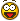##### Share on other sitesCheck this program...

(you have to be logged in...)

https://www.theswamp.org/index.php?topic=48113.msg531626#msg531626

Regards, M.R.

•1
##### Share on other sites
• 1 year later...Hello.

The script is not working anymore on Autocad 2020. On LSP load I get: "error: malformed list on input".

##### Share on other sitesthis error message is usually caused by a missing ) or ( so maybe you cut-copy-pasted to much? Maybe download file again from original link.

##### Share on other sites13 hours ago, cupax said:

Hello.

The script is not working anymore on Autocad 2020. On LSP load I get: "error: malformed list on input".

Edited by ronjonp
##### Share on other sitesYou mean after the 1e- ?

`(if (equal d (+ d1 d2) 1e-) (setq result T) (setq result nil))`

(I tested the version from the link provided by marko_ribar)

##### Share on other sites17 minutes ago, rlx said:

You mean after the 1e- ?

```
(if (equal d (+ d1 d2) 1e-) (setq result T) (setq result nil))```

(I tested the version from the link provided by marko_ribar)

Note - you also need to change the "-" in "1e-" to a number of decimal places like "1e6". Otherwise it does not work.

Edited by pkenewell
##### Share on other sitesoops... you're right pkenewell ... not sure how accurate it should be though , 1e-4 , 1e-8 or 1e-14... guess just a matter of trial and error. But good catch from both you and ronjonp`(if (equal d (+ d1 d2) 1e-4) (setq result T) (setq result nil))`
##### Share on other sitesMust be enough.`(setq result (if (equal d (+ d1 d2) 1e-4) T nil))`

##### Share on other sitesThe original would have been 1e-8 since all occurrences of "8)" were removed from all code posts across the forum when the forum software was "upgraded".

•1
##### Share on other sites3 hours ago, rlx said:

You mean after the 1e- ?

```
(if (equal d (+ d1 d2) 1e-) (setq result T) (setq result nil))```

(I tested the version from the link provided by marko_ribar)

That is correct .. I posted in haste. I'll correct above.

##### Share on other sitesThanks guys, now it works.

If I may suggest an enhancement: the script is missing the "awareness" of where it already placed an instance of the block. So if you are doing a tree forest, you are going to get too much trees overlapping - placed one above another. Not a very natural distribution.

There should be an option to define the invisible clearance circle around each instance.

## Join the conversation

You can post now and register later. If you have an account, sign in now to post with your account.
Note: Your post will require moderator approval before it will be visible.×   Pasted as rich text.   Restore formatting

Only 75 emoji are allowed.